# Mapped CI Engine

Compression-ignition engine model using lookup tables

• Library:
• Powertrain Blockset / Propulsion / Combustion Engines

Vehicle Dynamics Blockset / Powertrain / Propulsion

•## Description

The Mapped CI Engine block implements a mapped compression-ignition (CI) engine model using power, air mass flow, fuel flow, exhaust temperature, efficiency, and emission performance lookup tables. You can use the block for:

• Hardware-in-the-loop (HIL) engine control design

• Vehicle-level fuel economy and performance simulations

The lookup tables, developed with the Model-Based Calibration Toolbox™, are functions of injected fuel mass, F, engine torque, T, engine speed, N, and engine temperature, TempEng.

Setting

Parameter Setting

Lookup Tables
`Fuel mass``off`ƒ(F,N)
`on`ƒ(F,N,TempEng)
`Torque``off`ƒ(T,N)
`on`ƒ(T,N,TempEng)

The block enables you to specify lookup tables for these engine characteristics:

• Power

• Air

• Fuel

• Temperature

• Efficiency

• Hydrocarbon (HC) emissions

• Carbon monoxide (CO) emissions

• Nitric oxide and nitrogen dioxide (NOx) emissions

• Carbon dioxide (CO2) emissions

• Particulate matter (PM) emissions

To bound the Mapped CI Engine block output, the block does not extrapolate the lookup table data.

### Virtual Calibration

If you have Model-Based Calibration Toolbox, click Calibrate Maps to virtually calibrate the 2D lookup tables using measured data. The dialog box steps through these tasks.

Description

Import firing data

Import this loss data from a file. For example, open `<matlabroot>/toolbox/mbc/mbctraining/CiEngineData.xlsx`.

Input command

Required Data

Optional Data

`Fuel mass`
• Engine speed, rpm

• Commanded fuel mass per injection, mg

• Engine torque, N·m

• Air mass flow rate, kg/s

• Brake specific fuel consumption, g/(kW·h)

• CO2 mass flow rate, kg/s

• CO mass flow rate, kg/s

• Exhaust temperature, K

• Fuel mass flow rate, kg/s

• HC mass flow rate, kg/s

• NOx mass flow rate, kg/s

• Particulate matter mass flow rate, kg/s

`Torque`
• Engine speed, rpm

• Engine torque, N·m

Collect firing data at steady-state operating conditions when injectors deliver the fuel. Data should cover the engine speed and torque operating range. Model-Based Calibration Toolbox uses the firing data boundary as the maximum torque.

To filter or edit the data, select . The Model-Based Calibration Toolbox Data Editor opens.

Import non-firing data

Import this non-firing data from a file. For example, open `<matlabroot>/toolbox/autoblks/autodemos/projectsrc/CIDynamometer/CalMappedEng/CiEngineData.xlsx`.

• Engine speed, rpm

• Engine torque, N·m

Collect non-firing (motoring) data at steady-state operating conditions when fuel is cut off. All non-firing torque points must be less than zero. Non-firing data is a function of engine speed only.

Generate response models

For both firing and non-firing data, the Model-Based Calibration Toolbox uses test plans to fit data to Gaussian process models (GPMs).

To assess or adjust the response model fit, select . The Model-Based Calibration Toolbox Model Browser opens. For more information, see Model Assessment (Model-Based Calibration Toolbox).

Generate calibration

Model-Based Calibration Toolbox calibrates the firing and non-firing response models and generates calibrated tables.

To assess or adjust the calibration, select . The Model-Based Calibration Toolbox CAGE Browser opens. For more information, see Calibration Lookup Tables (Model-Based Calibration Toolbox).

Update block parameters

Update the block lookup table and breakpoint parameters with the calibration.

### Cylinder Air Mass

The block calculates the normalized cylinder air mass using these equations.

`$\begin{array}{l}{M}_{Nom}=\frac{{P}_{std}{V}_{d}}{{N}_{cyl}{R}_{air}{T}_{std}}\\ L=\frac{\left(\frac{60s}{min}\right)Cps\cdot {\stackrel{˙}{m}}_{air}}{\left(\frac{1000g}{Kg}\right){N}_{cyl}\cdot N\cdot {M}_{Nom}}\end{array}$`

The equations use these variables.

 L Normalized cylinder air mass ${M}_{Nom}$ Nominal engine cylinder air mass at standard temperature and pressure, piston at bottom dead center (BDC) maximum volume, in kg $Cps$ Crankshaft revolutions per power stroke, rev/stroke ${P}_{std}$ Standard pressure ${T}_{std}$ Standard temperature ${R}_{air}$ Ideal gas constant for air and burned gas mixture ${V}_{d}$ Displaced volume ${N}_{cyl}$ Number of engine cylinders N Engine speed ${\stackrel{˙}{m}}_{intk}$ Engine air mass flow, in g/s

### Turbocharger Lag

To model turbocharger lag, select Include turbocharger lag effect. Turbocharger lag limits the maximum fuel mass per injection. To model the maximum fuel mass per injection, the block uses a first-order system with a time constant. At low torque, the engine does not require boost to provide sufficient air flow. When the requested fuel mass requires boost, the block uses a time constant to determine the maximum fuel mass per injection. The block uses these equations for the specified Input command setting.

CalculationInput command Parameter Setting
`Fuel mass``Torque`

Dynamic torque

`$\frac{d{F}_{max}}{dt}=\frac{1}{{\tau }_{eng}}\left({F}_{cmd}-{F}_{max}\right)$`

`$\frac{d{T}_{max}}{dt}=\frac{1}{{\tau }_{eng}}\left({T}_{cmd}-{T}_{max}\right)$`

Fuel mass per injection or torque - with turbocharger lag

Fuel mass per injection or torque- without turbocharger lag

`$F={F}_{cmd}={F}_{max}$`

`${T}_{target}={T}_{cmd}={T}_{max}$`

Boost time constant

Final time constant

The equations use these variables.

 Tbrake Brake torque F Fuel mass per injection Fcmd, Fmax Commanded and maximum fuel mass per injection, respectively Ttarget, Tcmd, Tmax Target, commanded, and maximum torque, respectively τbst Boost time constant τbst,rising, τbst,falling Boost rising and falling time constant, respectively τeng Final time constant τnat Time constant below the boost torque speed line ƒbst(N) Boost torque/speed line N Engine speed

### Fuel Flow

To calculate the fuel economy for high-fidelity models, the block uses the volumetric fuel flow.

`${Q}_{fuel}=\frac{{\stackrel{˙}{m}}_{fuel}}{\left(\frac{1000kg}{{m}^{3}}\right)S{g}_{fuel}}$`

The equation uses these variables.

 ${\stackrel{˙}{m}}_{fuel}$ Fuel mass flow Sgfuel Specific gravity of fuel Qfuel Volumetric fuel flow

### Power Accounting

For the power accounting, the block implements these equations.

Bus Signal DescriptionEquations

`PwrInfo`

`PwrTrnsfrd` — Power transferred between blocks

• Positive signals indicate flow into block

• Negative signals indicate flow out of block

`PwrCrkshft`

Crankshaft power

$-{\tau }_{eng}\omega$

`PwrNotTrnsfrd` — Power crossing the block boundary, but not transferred

• Positive signals indicate an input

• Negative signals indicate a loss

`PwrFuel`

Fuel input power

${\stackrel{˙}{m}}_{fuel}LHV$

`PwrLoss`

Power loss

${\tau }_{eng}\omega -{\stackrel{˙}{m}}_{fuel}LHV$

`PwrStored` — Stored energy rate of change

• Positive signals indicate an increase

• Negative signals indicate a decrease

Not used

The equations use these variables.

 LHV Fuel lower heating value ω Engine speed, rad/s ${\stackrel{˙}{m}}_{fuel}$ Fuel mass flow τeng Fuel mass per injection time constant

## Ports

### Input

expand all

Injected fuel mass command, F, in mg/inj.

#### Dependencies

To enable this port, for Input command, select `Fuel mass`.

Torque command, T, in N·m.

#### Dependencies

To enable this port, for Input command, select `Torque`.

Engine speed, N, in rpm.

Engine temperature, TempEng, in K.

#### Dependencies

To enable this port, select Input engine temperature.

### Output

expand all

Bus signal containing these block calculations.

SignalDescriptionUnits

`IntkGasMassFlw`

Engine air mass flow output

kg/s

`NrmlzdAirChrg`

Normalized engine cylinder air mass

N/A

`Afr`

Air-fuel ratio (AFR)

N/A

`FuelMassFlw`

Engine fuel flow output

kg/s

`FuelVolFlw`

Volumetric fuel flow

m3/s

`ExhManGasTemp`

Engine exhaust gas temperature

K

`EngTrq`

Engine torque output

N·m

`EngSpd`

Engine speed

rpm

`CrkAng`

Engine crankshaft absolute angle

$\underset{0}{\overset{\left(360\right)Cps}{\int }}EngSpd\frac{180}{30}d\theta$

where $Cps$ is crankshaft revolutions per power stroke.

degrees crank angle

`Bsfc`

Engine brake-specific fuel consumption (BSFC)

g/kWh

`EoHC`

Engine out hydrocarbon emission mass flow

kg/s

`EoCO`

Engine out carbon monoxide emission mass flow rate

kg/s

`EoNOx`

Engine out nitric oxide and nitrogen dioxide emissions mass flow

kg/s

`EoCO2`

Engine out carbon dioxide emission mass flow

kg/s

`EoPM`

Engine out particulate matter emission mass flow

kg/s

`PwrInfo``PwrTrnsfrd``PwrCrkshft`

Crankshaft power

W
`PwrNotTrnsfrd``PwrFuel`

Fuel input power

W
`PwrLoss`

Power loss

W
`PwrStored`

Not used

Engine power, ${T}_{brake}$, in N·m.

## Parameters

expand all

Block Options

The lookup tables, developed with the Model-Based Calibration Toolbox, are functions of injected fuel mass, F, engine torque, T, engine speed, N, and engine temperature, TempEng.

Setting

Parameter Setting

Lookup Tables
`Fuel mass``off`ƒ(F,N)
`on`ƒ(F,N,TempEng)
`Torque``off`ƒ(T,N)
`on`ƒ(T,N,TempEng)

#### Dependencies

• Selecting `Fuel mass` enables Breakpoints for commanded fuel mass input, f_tbrake_f_bpt.

• Selecting `Torque` enables Breakpoints for commanded torque input, f_tbrake_t_bpt.

• Selecting Input engine temperature enables Breakpoints for temperature input, f_tbrake_engtmp_bpt.

To model turbocharger lag, select Include turbocharger lag effect. Turbocharger lag limits the maximum fuel mass per injection. To model the maximum fuel mass per injection, the block uses a first-order system with a time constant. At low torque, the engine does not require boost to provide sufficient air flow. When the requested fuel mass requires boost, the block uses a time constant to determine the maximum fuel mass per injection. The block uses these equations for the specified Input command setting.

CalculationInput command Parameter Setting
`Fuel mass``Torque`

Dynamic torque

`$\frac{d{F}_{max}}{dt}=\frac{1}{{\tau }_{eng}}\left({F}_{cmd}-{F}_{max}\right)$`

`$\frac{d{T}_{max}}{dt}=\frac{1}{{\tau }_{eng}}\left({T}_{cmd}-{T}_{max}\right)$`

Fuel mass per injection or torque - with turbocharger lag

Fuel mass per injection or torque- without turbocharger lag

`$F={F}_{cmd}={F}_{max}$`

`${T}_{target}={T}_{cmd}={T}_{max}$`

Boost time constant

Final time constant

The equations use these variables.

 Tbrake Brake torque F Fuel mass per injection Fcmd, Fmax Commanded and maximum fuel mass per injection, respectively Ttarget, Tcmd, Tmax Target, commanded, and maximum torque, respectively τbst Boost time constant τbst,rising, τbst,falling Boost rising and falling time constant, respectively τeng Final time constant τnat Time constant below the boost torque speed line ƒbst(N) Boost torque/speed line N Engine speed

#### Dependencies

Selecting Include turbocharger lag effect enables these parameters:

• Boost torque line, f_tbrake_bst

• Time constant below boost line, tau_nat

• Rising maximum fuel mass boost time constant, tau_bst_rising

• Falling maximum fuel mass boost time constant, tau_bst_falling

Select this to create the `EngTemp` input port.

The lookup tables, developed with the Model-Based Calibration Toolbox, are functions of injected fuel mass, F, engine torque, T, engine speed, N, and engine temperature, TempEng.

Setting

Parameter Setting

Lookup Tables
`Fuel mass``off`ƒ(F,N)
`on`ƒ(F,N,TempEng)
`Torque``off`ƒ(T,N)
`on`ƒ(T,N,TempEng)

Configuration

If you have Model-Based Calibration Toolbox, click Calibrate Maps to virtually calibrate the 2D lookup tables using measured data. The dialog box steps through these tasks.

Description

Import firing data

Import this loss data from a file. For example, open `<matlabroot>/toolbox/mbc/mbctraining/CiEngineData.xlsx`.

Input command

Required Data

Optional Data

`Fuel mass`
• Engine speed, rpm

• Commanded fuel mass per injection, mg

• Engine torque, N·m

• Air mass flow rate, kg/s

• Brake specific fuel consumption, g/(kW·h)

• CO2 mass flow rate, kg/s

• CO mass flow rate, kg/s

• Exhaust temperature, K

• Fuel mass flow rate, kg/s

• HC mass flow rate, kg/s

• NOx mass flow rate, kg/s

• Particulate matter mass flow rate, kg/s

`Torque`
• Engine speed, rpm

• Engine torque, N·m

Collect firing data at steady-state operating conditions when injectors deliver the fuel. Data should cover the engine speed and torque operating range. Model-Based Calibration Toolbox uses the firing data boundary as the maximum torque.

To filter or edit the data, select . The Model-Based Calibration Toolbox Data Editor opens.

Import non-firing data

Import this non-firing data from a file. For example, open `<matlabroot>/toolbox/autoblks/autodemos/projectsrc/CIDynamometer/CalMappedEng/CiEngineData.xlsx`.

• Engine speed, rpm

• Engine torque, N·m

Collect non-firing (motoring) data at steady-state operating conditions when fuel is cut off. All non-firing torque points must be less than zero. Non-firing data is a function of engine speed only.

Generate response models

For both firing and non-firing data, the Model-Based Calibration Toolbox uses test plans to fit data to Gaussian process models (GPMs).

To assess or adjust the response model fit, select . The Model-Based Calibration Toolbox Model Browser opens. For more information, see Model Assessment (Model-Based Calibration Toolbox).

Generate calibration

Model-Based Calibration Toolbox calibrates the firing and non-firing response models and generates calibrated tables.

To assess or adjust the calibration, select . The Model-Based Calibration Toolbox CAGE Browser opens. For more information, see Calibration Lookup Tables (Model-Based Calibration Toolbox).

Update block parameters

Update the block lookup table and breakpoint parameters with the calibration.

#### Dependencies

To enable this parameter, clear Input engine temperature.

Breakpoints, in mg/inj.

#### Dependencies

Setting Input command to ```Fuel mass``` enables this parameter.

Breakpoints, in N·m.

#### Dependencies

Setting Input command to `Torque` enables this parameter.

Breakpoints, in rpm.

Breakpoints, in K.

#### Dependencies

To enable this parameter, select Input engine temperature.

Number of cylinders.

Crank revolutions per power stroke.

Volume displaced by engine, in m^3.

Fuel lower heating value, LHV, in J/kg.

Specific gravity of fuel, Sgfuel, dimensionless.

Ideal gas constant of air and residual gas entering the engine intake port, in J/(kg·K).

Standard air pressure, in Pa.

Standard air temperature, in K.

Boost torque line, ƒbst(N), in N·m.

#### Dependencies

To enable this parameter, select Include turbocharger lag effect.

Time constant below boost line, τnat, in s.

#### Dependencies

To enable this parameter, select Include turbocharger lag effect.

Rising maximum fuel mass boost time constant, τbst,rising, in s.

#### Dependencies

To enable this parameter, select Include turbocharger lag effect.

Falling maximum fuel mass boost time constant, τbst,falling, in s.

#### Dependencies

To enable this parameter, select Include turbocharger lag effect.

Turbocharger time constant blend fuel mass fraction, in s.

#### Dependencies

To enable this parameter, select Include turbocharger lag effect.

Power
Input Command SettingDescription
`Fuel mass`

The engine brake torque lookup table is a function of commanded fuel mass and engine speed, ${T}_{brake}$ = ƒ(F, N), where:

• ${T}_{brake}$ is engine torque, in N·m.

• F is commanded fuel mass, in mg per injection.

• N is engine speed, in rpm.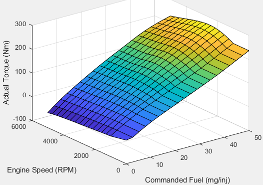`Torque`

The engine brake torque lookup table is a function of target torque and engine speed, ${T}_{brake}$ = ƒ(Ttarget, N), where:

• ${T}_{brake}$ is engine torque, in N·m.

• Ttarget is target torque, in N·m.

• N is engine speed, in rpm.

#### Dependencies

To enable this parameter, clear Input engine temperature.

Click to plot table.

#### Dependencies

To enable this parameter, clear Input engine temperature.

Input Command SettingDescription
`Fuel mass`

The engine brake torque lookup table is a function of commanded fuel mass and engine speed, ${T}_{brake}$ = ƒ(F, N, TempEng), where:

• ${T}_{brake}$ is engine torque, in N·m.

• F is commanded fuel mass, in mg per injection.

• TempEng is engine temperature, in K.

`Torque`

The engine brake torque lookup table is a function of target torque and engine speed, ${T}_{brake}$ = ƒ(Ttarget, N, TempEng), where:

• ${T}_{brake}$ is engine torque, in N·m.

• Ttarget is target torque, in N·m.

• N is engine speed, in rpm.

• TempEng is engine temperature, in K.

#### Dependencies

To enable this parameter, select Input engine temperature.

Air
Input Command SettingDescription
`Fuel mass`

The air mass flow lookup table is a function of commanded fuel mass and engine speed, ${\stackrel{˙}{m}}_{intk}$ = ƒ(Fmax, N), where:

• ${\stackrel{˙}{m}}_{intk}$ is engine air mass flow, in kg/s.

• Fmax is commanded fuel mass, in mg per injection.

• N is engine speed, in rpm.`Torque`

The air mass flow lookup table is a function of maximum torque and engine speed, ${\stackrel{˙}{m}}_{intk}$ = ƒ(Tmax, N), where:

• ${\stackrel{˙}{m}}_{intk}$ is engine air mass flow, in kg/s.

• Tmax is maximum torque, in N·m.

• N is engine speed, in rpm.

#### Dependencies

To enable this parameter, clear Input engine temperature.

Click to plot table.

#### Dependencies

To enable this parameter, clear Input engine temperature.

Input Command SettingDescription
`Fuel mass`

The air mass flow lookup table is a function of commanded fuel mass and engine speed, ${\stackrel{˙}{m}}_{intk}$ = ƒ(Fmax, N, TempEng), where:

• ${\stackrel{˙}{m}}_{intk}$ is engine air mass flow, in kg/s.

• Fmax is commanded fuel mass, in mg per injection.

• N is engine speed, in rpm.

• TempEng is engine temperature, in K.

`Torque`

The air mass flow lookup table is a function of maximum torque and engine speed, ${\stackrel{˙}{m}}_{intk}$ = ƒ(Tmax, N, TempEng), where:

• ${\stackrel{˙}{m}}_{intk}$ is engine air mass flow, in kg/s.

• Tmax is maximum torque, in N·m.

• N is engine speed, in rpm.

• TempEng is engine temperature, in K.

#### Dependencies

To enable this parameter, select Input engine temperature.

Fuel
Input Command SettingDescription
`Fuel mass`

The engine fuel flow lookup table is a function of commanded fuel mass and engine speed, MassFlow= ƒ(F, N), where:

• MassFlow is engine fuel mass flow, in kg/s.

• F is commanded fuel mass, in mg per injection.

• N is engine speed, in rpm.`Torque`

The engine fuel flow lookup table is a function of target torque and engine speed, MassFlow = ƒ(Ttarget, N), where:

• MassFlow is engine fuel mass flow, in kg/s.

• Ttarget is target torque, in N·m.

• N is engine speed, in rpm.

#### Dependencies

To enable this parameter, clear Input engine temperature.

Click to plot table.

#### Dependencies

To enable this parameter, clear Input engine temperature.

Input Command SettingDescription
`Fuel mass`

The engine fuel flow lookup table is a function of commanded fuel mass, engine speed, and engine temperature, MassFlow= ƒ(F, N, TempEng), where:

• MassFlow is engine fuel mass flow, in kg/s.

• F is commanded fuel mass, in mg per injection.

• N is engine speed, in rpm.

• TempEng is engine temperature, in K.

`Torque`

The engine fuel flow lookup table is a function of target torque and engine speed, and engine temperature, MassFlow = ƒ(Ttarget, N, TempEng), where:

• MassFlow is engine fuel mass flow, in kg/s.

• Ttarget is target torque, in N·m.

• N is engine speed, in rpm.

• TempEng is engine temperature, in K.

#### Dependencies

To enable this parameter, select Input engine temperature.

Temperature
Input Command SettingDescription
`Fuel mass`

The engine exhaust temperature table is a function of commanded fuel mass and engine speed, Texh= ƒ(F, N), where:

• Texhis exhaust temperature, in K.

• F is commanded fuel mass, in mg per injection.

• N is engine speed, in rpm.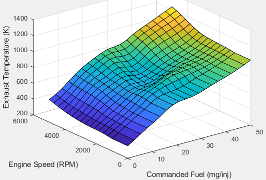`Torque`

The engine exhaust temperature table is a function of target torque and engine speed, Texh = ƒ(Ttarget, N), where:

• Texh is exhaust temperature, in K.

• Ttarget is target torque, in N·m.

• N is engine speed, in rpm.

#### Dependencies

To enable this parameter, clear Input engine temperature.

Click to plot table.

#### Dependencies

To enable this parameter, clear Input engine temperature.

Input Command SettingDescription
`Fuel mass`

The engine exhaust temperature table is a function of commanded fuel mass and engine speed, Texh= ƒ(F, N, TempEng), where:

• Texhis exhaust temperature, in K.

• F is commanded fuel mass, in mg per injection.

• N is engine speed, in rpm.

• TempEng is engine temperature, in K.

`Torque`

The engine exhaust temperature table is a function of target torque and engine speed, Texh = ƒ(Ttarget, N, TempEng), where:

• Texh is exhaust temperature, in K.

• Ttarget is target torque, in N·m.

• N is engine speed, in rpm.

• TempEng is engine temperature, in K.

#### Dependencies

To enable this parameter, select Input engine temperature.

Efficiency
Input Command SettingDescription
`Fuel mass`

The brake-specific fuel consumption (BSFC) efficiency is a function of commanded fuel mass and engine speed, BSFC= ƒ(F, N), where:

• BSFC is BSFC, in g/kWh.

• F is commanded fuel mass, in mg per injection.

• N is engine speed, in rpm.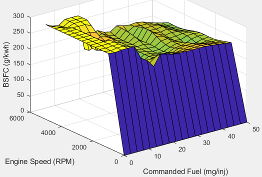`Torque`

The brake-specific fuel consumption (BSFC) efficiency is a function of target torque and engine speed, BSFC = ƒ(Ttarget, N), where:

• BSFC is BSFC, in g/kWh.

• Ttarget is target torque, in N·m.

• N is engine speed, in rpm.

#### Dependencies

To enable this parameter, clear Input engine temperature.

Click to plot table.

#### Dependencies

To enable this parameter, clear Input engine temperature.

Input Command SettingDescription
`Fuel mass`

The brake-specific fuel consumption (BSFC) efficiency is a function of commanded fuel mass and engine speed, BSFC= ƒ(F, N, TempEng), where:

• BSFC is BSFC, in g/kWh.

• F is commanded fuel mass, in mg per injection.

• N is engine speed, in rpm.

• TempEng is engine temperature, in K.

`Torque`

The brake-specific fuel consumption (BSFC) efficiency is a function of target torque and engine speed, BSFC = ƒ(Ttarget, N, TempEng), where:

• BSFC is BSFC, in g/kWh.

• Ttarget is target torque, in N·m.

• N is engine speed, in rpm.

• TempEng is engine temperature, in K.

#### Dependencies

To enable this parameter, select Input engine temperature.

HC
Input Command SettingDescription
`Fuel mass`

The engine-out hydrocarbon emissions are a function of commanded fuel mass and engine speed, EO HC= ƒ(F, N), where:

• EO HC is engine-out hydrocarbon emissions, in kg/s.

• F is commanded fuel mass, in mg per injection.

• N is engine speed, in rpm.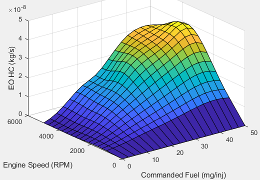`Torque`

The engine-out hydrocarbon emissions are a function of target torque and engine speed, EO HC = ƒ(Ttarget, N), where:

• EO HC is engine-out hydrocarbon emissions, in kg/s.

• Ttarget is target torque, in N·m.

• N is engine speed, in rpm.

#### Dependencies

To enable this parameter, clear Input engine temperature.

Click to plot table.

#### Dependencies

To enable this parameter, clear Input engine temperature.

Input Command SettingDescription
`Fuel mass`

The engine-out hydrocarbon emissions are a function of commanded fuel mass and engine speed, EO HC= ƒ(F, N, TempEng), where:

• EO HC is engine-out hydrocarbon emissions, in kg/s.

• F is commanded fuel mass, in mg per injection.

• N is engine speed, in rpm.

• TempEng is engine temperature, in K.

`Torque`

The engine-out hydrocarbon emissions are a function of target torque and engine speed, EO HC = ƒ(Ttarget, N, TempEng), where:

• EO HC is engine-out hydrocarbon emissions, in kg/s.

• Ttarget is target torque, in N·m.

• N is engine speed, in rpm.

• TempEng is engine temperature, in K.

#### Dependencies

To enable this parameter, select Input engine temperature.

CO
Input Command SettingDescription
`Fuel mass`

The engine-out carbon monoxide emissions are a function of commanded fuel mass and engine speed, EO CO= ƒ(F, N), where:

• EO CO is engine-out carbon monoxide emissions, in kg/s.

• F is commanded fuel mass, in mg per injection.

• N is engine speed, in rpm.`Torque`

The engine-out carbon monoxide emissions are a function of target torque and engine speed, EO CO = ƒ(Ttarget, N), where:

• EO CO is engine-out carbon monoxide emissions, in kg/s.

• Ttarget is target torque, in N·m.

• N is engine speed, in rpm.

#### Dependencies

To enable this parameter, clear Input engine temperature.

Click to plot table.

#### Dependencies

To enable this parameter, clear Input engine temperature.

Input Command SettingDescription
`Fuel mass`

The engine-out carbon monoxide emissions are a function of commanded fuel mass and engine speed, EO CO= ƒ(F, N, TempEng), where:

• EO CO is engine-out carbon monoxide emissions, in kg/s.

• F is commanded fuel mass, in mg per injection.

• N is engine speed, in rpm.

• TempEng is engine temperature, in K.

`Torque`

The engine-out carbon monoxide emissions are a function of target torque and engine speed, EO CO = ƒ(Ttarget, N, TempEng), where:

• EO CO is engine-out carbon monoxide emissions, in kg/s.

• Ttarget is target torque, in N·m.

• N is engine speed, in rpm.

• TempEng is engine temperature, in K.

#### Dependencies

To enable this parameter, select Input engine temperature.

NOx
Input Command SettingDescription
`Fuel mass`

The engine-out nitric oxide and nitrogen dioxide emissions are a function of commanded fuel mass and engine speed, EO NOx= ƒ(F, N), where:

• EO NOx is engine-out nitric oxide and nitrogen dioxide emissions, in kg/s.

• F is commanded fuel mass, in mg per injection.

• N is engine speed, in rpm.`Torque`

The engine-out nitric oxide and nitrogen dioxide emissions are a function of target torque and engine speed, EO NOx = ƒ(Ttarget, N), where:

• EO NOx is engine-out nitric oxide and nitrogen dioxide emissions, in kg/s.

• Ttarget is target torque, in N·m.

• N is engine speed, in rpm.

#### Dependencies

To enable this parameter, clear Input engine temperature.

Click to plot table.

#### Dependencies

To enable this parameter, clear Input engine temperature.

Input Command SettingDescription
`Fuel mass`

The engine-out nitric oxide and nitrogen dioxide emissions are a function of commanded fuel mass, engine speed, and engine temperature, EO NOx= ƒ(F, N, TempEng), where:

• EO NOx is engine-out nitric oxide and nitrogen dioxide emissions, in kg/s.

• F is commanded fuel mass, in mg per injection.

• N is engine speed, in rpm.

• TempEng is engine temperature, in K.

`Torque`

The engine-out nitric oxide and nitrogen dioxide emissions are a function of target torque, engine speed, and engine temperature, EO NOx = ƒ(Ttarget, N, TempEng), where:

• EO NOx is engine-out nitric oxide and nitrogen dioxide emissions, in kg/s.

• Ttarget is target torque, in N·m.

• N is engine speed, in rpm.

• TempEng is engine temperature, in K.

#### Dependencies

To enable this parameter, select Input engine temperature.

CO2
Input Command SettingDescription
`Fuel mass`

The engine-out carbon dioxide emissions are a function of commanded fuel mass and engine speed, EO CO2= ƒ(F, N), where:

• EO CO2 is engine-out carbon dioxide emissions, in kg/s.

• F is commanded fuel mass, in mg per injection.

• N is engine speed, in rpm.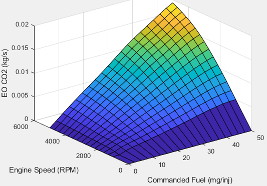`Torque`

The engine-out carbon dioxide emissions are a function of target torque and engine speed, EO CO2 = ƒ(Ttarget, N), where:

• EO CO2 is engine-out carbon dioxide emissions, in kg/s.

• Ttarget is target torque, in N·m.

• N is engine speed, in rpm.

#### Dependencies

To enable this parameter, clear Input engine temperature.

Click to plot table.

#### Dependencies

To enable this parameter, clear Input engine temperature.

Input Command SettingDescription
`Fuel mass`

The engine-out carbon dioxide emissions are a function of commanded fuel mass, engine speed, and engine temperature, EO CO2= ƒ(F, N, TempEng), where:

• EO CO2 is engine-out carbon dioxide emissions, in kg/s.

• F is commanded fuel mass, in mg per injection.

• N is engine speed, in rpm.

• TempEng is engine temperature, in K.

`Torque`

The engine-out carbon dioxide emissions are a function of target torque, engine speed, and engine temperature, EO CO2 = ƒ(Ttarget, N, TempEng), where:

• EO CO2 is engine-out carbon dioxide emissions, in kg/s.

• Ttarget is target torque, in N·m.

• N is engine speed, in rpm.

• TempEng is engine temperature, in K.

#### Dependencies

To enable this parameter, select Input engine temperature.

PM
Input Command SettingDescription
`Fuel mass`

The engine-out PM emissions are a function of commanded fuel mass and engine speed, where:

• EO PM is engine-out PM emissions, in kg/s.

• F is commanded fuel mass, in mg per injection.

• N is engine speed, in rpm.

`Torque`

The engine-out PM emissions are a function of target torque and engine speed, EO PM = ƒ(Ttarget, N), where:

• EO PM is engine-out PM emissions, in kg/s.

• Ttarget is target torque, in N·m.

• N is engine speed, in rpm.

#### Dependencies

To enable this parameter, clear Input engine temperature.

Click to plot table.

#### Dependencies

To enable this parameter, clear Input engine temperature.

Input Command SettingDescription
`Fuel mass`

The engine-out PM emissions are a function of commanded fuel mass, engine speed, and engine temperature, where:

• EO PM is engine-out PM emissions, in kg/s.

• F is commanded fuel mass, in mg per injection.

• N is engine speed, in rpm.

• TempEng is engine temperature, in K.

`Torque`

The engine-out PM emissions are a function of target torque, engine speed, and engine temperature, EO PM = ƒ(Ttarget, N, T), where:

• EO PM is engine-out PM emissions, in kg/s.

• Ttarget is target torque, in N·m.

• N is engine speed, in rpm.

• TempEng is engine temperature, in K.

#### Dependencies

To enable this parameter, select Input engine temperature.

## Version History

Introduced in R2017a